# 2.6: Numerical ODE solving in Excel- Euler’s method, Runge Kutta, Dead time in ODE solving

•• Contributed by Peter Woolf et al.
• Assistant Professor (Chemical Engineering) at University of Michigan

Authors: (Presented: 9/8/06 /Date Revised: 9/19/06) Aaron Bennick, Bradley Anderson, Michael Salciccioli

Stewards: (9/5/07) Sean Gant, Jay Lee, Lance Dehne, Kelly Martin

## 6.1 Introduction

This article focuses on the modeling of ordinary differential equations (ODEs) of the form:

$\frac{d y}{d x}=f(x, y)$

In creating a model, a new value $$y_{i + 1}$$ is generated using the old or initial value yi, the slope estimate φ, and the step size h. This general formula can be applied in a stepwise fashion to model the solution. All stepwise models will take the following general form:

$y_{i+1}=y_{i}+\phi h$

The modeling methods discussed in this article are Euler’s method and the Runge-Kutta methods. The difference between the two methods is the way in which the slope φ is estimated.

### Choosing The Right Model and Step Size

The proper numerical modeling method heavily depends on the situation, the available resources, and the desired accuracy of the result. If only a quick estimate of a differential equation is required, the Euler method may provide the simplest solution. If much higher accuracy is required, a fifth-order Runge-Kutta method may be used. Engineers today, with the aid of computers and excel, should be capable of quickly and accurately estimating the solution to ODEs using higher-order Runge-Kutta methods.

In all numerical models, as the step size is decreased, the accuracy of the model is increased. The tradeoff here is that smaller step sizes require more computation and therefore increase the amount of time to obtain a solution. A balance between desired accuracy and time required for producing an answer can be achieved by selecting an appropriate step size. One suggested algorithm for selecting a suitable step size is to produce models using two different methods (possibly a second and third order Runge-Kutta). The steps size can then be systematically cut in half until the difference between both models is acceptably small (effectively creating an error tolerance).

## 6.2 Euler's Method

Euler's method is a simple one-step method used for solving ODEs. In Euler’s method, the slope, φ, is estimated in the most basic manner by using the first derivative at xi. This gives a direct estimate, and Euler’s method takes the form of

$y_{i+1}=y_{i}+f\left(x_{i}, y_{i}\right) h$

For demonstration, we will use the basic differential equation $$\frac{d y}{d x}=3 x^{2}+2 x+1$$ with the initial condition y(0) = 1. If a step size, h, is taken to be 0.5 over the interval 0 to 2, the solutions can be calculated as follows:

x

y_Euler

y_Actual

Error

y(0)

1

1

0

y(0.5)

1 + [3(0)^2 + 2(0) + 1](0.5) = 1.5

1.875

0.375

y(1)

1.5 + [3(0.5)^2 + 2(0.5) + 1](0.5) = 2.875

4

1.125

y(1.5)

2.875 + [3(1)^2 + 2(1) + 1](0.5) = 5.875

8.125

3.25

y(2.0)

5.875 + [3(1.5)^2 + 2(1.5) + 1](0.5) = 11.25

15

3.75

The y_actual values in this table were calculated by directly integrating the differential equation, giving the exact solution as: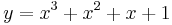### 6.2.1 Use of Microsoft Excel

Calculating an ODE solution by hand with Euler's method can be a very tedious process. Fortunately, this process is greatly simplified through the use of Microsoft Excel. Creating a spreadsheet similar to the one above, where the x values are specified and the y_Euler values are recursively calculated from the previous value, makes the calculation rather simple. Any step size and interval can be used.

### 6.2.2 Drawbacks of Euler's Method

There are several drawbacks to using Euler’s method for solving ODEs that must be kept in mind. First of all, this method does not work well on stiff ODEs. A stiff ODE is a differential equation whose solutions are numerically unstable when solved with certain numerical methods. For stiff equations - which are frequently encountered in modeling chemical kinetics - explicit methods like Euler's are usually quite inefficient because the region of stability is so small that the step size must be extremely small to get any accuracy. In a case like this, an implicit method, such as the backwards Euler method, yields a more accurate solution. These implicit methods require more work per step, but the stability region is larger. This allows for a larger step size, making the overall process more efficient than an explicit method. A second drawback to using Euler's Method is that error is introduced into the solution. The error associated with the simple example above is shown in the last column. This error can be seen visually in the graph below.It can be seen that the two values are identical at the initial condition of y(0)=1, and then the error increases as the x value increases and the error propagates through the solution to x = 2. The error can be decreased by choosing a smaller step size, which can be done quite easily in Excel, or by opting to solve the ODE with the more accurate Runge-Kutta method.

## 6.3 Runge-Kutta Methods

The Runge-Kutta method for modeling differential equations builds upon the Euler method to achieve a greater accuracy. Multiple derivative estimates are made and, depending on the specific form of the model, are combined in a weighted average over the step interval. The order of the Runge-Kutta method can range from second to higher, depending on the amount of derivative estimates made. The second-order Runge-Kutta method labeled Heun's technique estimates derivatives by averaging endpoint measurements of the step size along a function. This averaged value is used as the slope estimate for xi + 1. Third and higher power Runge-Kutta methods make mid-point derivative estimations, and deliver a weighted average for the end point derivative at xi + 1. As the Runge-Kutta order increases, so does the accuracy of the model.

The general form of the Runge-Kutta method is

$y_{i+1}=y_{i}+\phi\left(x_{i}, y_{i}, h\right) h$

Where $$φ(x_i,y_i,h)$$ now represents a weighted average slope over the interval $$h$$.

$\phi\left(x_{i}, y_{i}, h\right)=a_{1} k_{1}+a_{2} k_{2}+\ldots+a_{n} K_{n}$

where the a's are constants and the k's areThe constants a, p, and q are solved for with the use of Taylor series expansions once n is specified (see bottom of page for derivation). The resulting set of equations have one or more degrees of freedom. This means that for every order of Runge-Kutta method, there is a family of methods. Below are some of the more common Runge-Kutta choices.

### 6.3.1 Second-Order Runge-Kutta Methods (n = 2)

Every second order method described here will produce exactly the same result if the modeled differential equation is constant, linear, or quadratic. Because this is typically not the case, and the differential equation is often more complicated, one method may be more suitable than another.

#### Heun's Technique

The second-order Runge-Kutta method with one iteration of the slope estimate , also known as Heun's technique, sets the constants

$a_1=a_2=\ce{1/2}$

and

$p_1=q_{11}=1.$

Huen determined that defining $$a_1$$ and $$a_2$$ as $$1/2$$ will take the average of the slopes of the tangent lines at either end of the desired interval, accounting for the concavity of the function, creating a more accurate result. When substituted into the general form, we find

$y_{i+1}=y_{i}+\left(\frac{1}{2} k_{1}+\frac{1}{2} k_{2}\right) h$

with k1 and k2 defined as

$k_{1}=f\left(x_{i}, y_{i}\right)$

$k_{2}=f\left(x_{i}+h, y_{i}+h k_{1}\right)$

For demonstration of this second-order Runge-Kutta method, we will use the same basic differential equation $$\frac{d y}{d x}=3 x^{2}+2 x+1$$ with the initial condition y(0) = 1. If a step size, h, is taken to be 0.5 over the interval 0 to 2, the solutions can be calculated as follows:

Figure 1: second-order Runge-Kutta

x

k_1

k_2

y_Heun

y_Actual

Error

y(0)

3(0)^2 + 2(0) + 1 = 1

3(0.5)^2 + 2(0.5) + 1 = 2.75

1

1

0

y(0.5)

3(0.5)^2 + 2(0.5) + 1 = 2.75

3(1)^2 + 2(1) + 1 = 6

1 + [0.5(1) + 0.5(2.75)](0.5) = 1.9375

1.875

-0.0625

y(1)

3(1)^2 + 2(1) + 1 = 6

3(1.5)^2 + 2(1.5) + 1 = 10.75

2.375 + [0.5(2.75) + 0.5(6)](0.5) = 4.125

4

-0.125

y(1.5)

3(1.5)^2 + 2(1.5) + 1 = 10.75

3(2)^2 + 2(2) + 1 = 17

5.375 + [0.5(6) + 0.5(10.75)](0.5) = 8.3125

8.125

-0.1875

y(2.0)

3(2)^2 + 2(2) + 1 = 17

3(2.5)^2 + 2(2.5) + 1 = 24.75

10.75 + [0.5(10.75) + 0.5(17)](0.5) = 15.25

15

-0.25

When compared to the Euler method demonstration above, it can be seen that the second-order Runge-Kutta Heun's Technique requires a significant increase in effort in order to produce values, but also produces a significant reduction in error. Following Runge-Kutta methods can be worked through a similar manner, adding columns for additional k values. Below is a graphical description of how slope is estimated using both Euler's method, and Heun's technique. Observe the increase in accuracy when an average slope across an interval of 0.5 is used instead of just an initial estimate.#### Improved Polygon Method

Using the improved polygon method, a2 is taken to be 1, a1 as 0, and therefore. The general form then becomeswith k1 and k2 defined asRalston's Method

The Ralston method takes a2 to be. Thereforeand. It has been determined by Ralston (1962) and Ralston and Rabinowitz (1978) that defining a2 aswill minimize truncation error in second-order Runge-Kutta methods. The general form becomeswith k1 and k2 defined as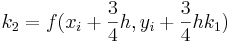### 6.3.2 Third-Order Runge-Kutta Methods (n = 3)

The third-order Runge-Kutta methods, when derived, produce a family of equations to solve for constants with two degrees of freedom. This means an even more variable family of third-order Runge-Kutta methods can be produced. A commonly used general third-order form iswith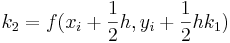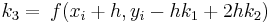### 6.3.3 Fourth-Order Runge-Kutta Methods (n = 4)

The family of fourth-order Runge-Kutta methods have three degrees of freedmon and therefore infinite variability just as the second and third order methods do. The fourth-order versions are most favored among all the Runge-Kutta methods. What is know as the classical fourth-order Runge-Kutta method is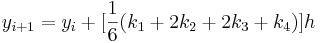with### 6.3.4 Fifth-Order Runge-Kutta Methods (n = 5)

Where very accurate results are required, the fifth-order Runge-Kutta Butcher's (1964) fifth-order RK method should be employed:withThe integration of k's within other k values suggests the use of a spreadsheet. As with all Runge-Kutta methods, the calculation of values for the fifth-order version would be greatly assisted through the use of Microsoft Excel.

## 6.4 Interactive Model Comparison in Excel

To give a better understanding of the impact between different Runge-Kutta methods, as well as the impact of step size, the interactive excel sheet below will allow you to enter the step size h into a set of models and observe how the models contour to match an example differential equation solution.

Observe the relationship between model type, step size, and relative error.

Dead time, or delay differential equation, occurs when there is a delay or lag in the process of a real life function that is being modeled. This means that the numerical model is not accurate until the delay is over. This concept can come into play for the start up of a reaction process. For example, at the start of a reaction in a CSTR (Continuous Stirred Tank Reactor), there will be reagents at the top of the reactor that have started the reaction, but it will take a given time for these reactant/products to be discharged from the reactor. If one was modeling the concentration of reagents vs. time, time t=0 would have started when the tank was filled, but the concentrations being read would not follow a standard model equation until the residence time was completed and the reactor was in continuous operational mode. The dead time is the time it would take for the readings to start meeting a theoretical equation, or the time it takes for the reactor to be cleared once of the original reagents. Dead time can be determined experimentally and then inserted into modeling equations.

The solution of such a delay differential equation becomes problematic because in order to solve the equation, information from past times (during the delay) is needed in addition to the current time. For example if to is the lag time for the given scenario, then the value to use in the ODE becomes (t-to) instead of t. When modeling this in excel, (x-to) is substituted in for the x value. With this substitution, Euler’s method can be used again in the same way to approximate the solution. This substitution will be carried into the differential equation so that the new dead time solution can be approximated using Euler's method. To model the reactor before the initial deadtime is completed, piece-wise functions are often used. This way for the dead time, a given model is used not characteristic of the reactor at normal operating conditions, then once the dead time is completed the modeling equation is taken into effect.

The link below will help to show how to include dead time in a numerical method approximation such as Euler's method. As seen in the excel file, the dead time that is specified by the user in the yellow box will change the delay in the model. The more dead time, the further shifted from the theoretical equation the new model is. To take dead time into account in excel, the x value is simply substituted out for (x-t) where t is equal to the dead time. If you look at the equations entered in the Y cells, you will see that the x value inserted into the differential equation is (x-t), where t is the user specified dead time. It can be seen through this example spreadsheet that the effect of dead time is a simple horizontal shift in the model equation.

## 6.6 Error

There are two types of error associated with solving ODEs using stepwise approximation methods in Excel. These errors are also present using other methods or computer programs to solve ODEs. The first, discretization, is the result of the estimated y value that is inherent of using a numerical method to approximate a solution. Discretization errors, also called truncation, occur proportionately over a single step size. Truncation error will propagate over extended results because the approximation from previous steps is used to approximate the next. In essence, a copy of a copy is being made. The accuracy of the next point is a direct result of the accuracy of the previous. Just as the quality of a second copy is dependant on the quality of the first. This error can be reduced by reducing the step size.

Please see ODE model comparison interactive spreadsheet to better learn how step sizes can influence error.

The second types of errors are rounding errors. These errors are dependent on the computer’s capacity for retaining significant digits. The more significant digits that a computer can hold, the smaller the rounding error will be.

#### 6.6.1 Estimating Error in Euler's Method

To mathematically represent the error associated with Euler's method, it is first helpful to make a comparison to an infinite Taylor series expansion of the term yi + 1. The Taylor series expansion of this term is

$y_{i+1}=y_{i}+f\left(x_{i}, y_{i}\right) \hbar+f^{\prime}\left(x_{i}, y_{i}\right) \frac{h^{2}}{2}+f^{\prime \prime}\left(x_{i}, y_{i}\right) \frac{h^{3}}{3}+\ldots+f^{n}\left(x_{i}, y_{i}\right) \frac{h^{n}}{! n}$

When this expansion is compared to the general form of Euler's method it can be seen that Euler's method lacks every term beyond. These missing terms, the difference between the Euler approximation and an infinite Taylor series (taken to be the true solution), is the error in the Euler approximation. Mathematically respresenting the error in higher order Runge-kutta methods is done in a similar fashion.

#### 6.6.2 Estimating and Minimizing Error in Runge Kutta Method

Uniform time steps are good for some cases but not always. Sometimes we deal with problems where varying time steps makes sense. When should you change the step size? If we have an nth order scheme and and (n+1)th order scheme, we can take the difference between these two to be the error in the scheme, and make the step size smaller if we prefer a smaller error, or larger if we can tolerate a larger error. This is fairly simple with Runge Kutta, because we can take a fifth order method and a fourth order method using the same k's. Only a little extra work at each step.

Another way of estimating error in the Runge-Kutta method is to reverse directions at each step of the advancing solution and recompute the previous ordinate. By considering the difference between the newly computed previous ordinate and the originally computed value, you can determine an estimate for the truncation error incurred in advancing the solution over that step. This is a bit more tedious, but does give a good estimate of truncation error.

## 6.7 Euler's Method for Systems of ODEs

In chemical engineering and other related fields, having a method for solving a differential equation is simply not enough. Many real world problems require simultaneously solving systems of ODEs. For problems like these, any of the numerical methods described in this article will still work. The only difference is that for n ODEs, n initial values of y are needed for the initial x value. Then the numerical method of choice is just applied to every equation in each step before going on to the next. This further complicates the step-by-step problem solving methodology, and would require the use of Excel in nearly every application. The ODE solved with Euler's method as an example before is now expanded to include a system of two ODEs below:

$\frac{d y_{1}}{d x}=3 x^{2}+2 x+1, y_{1}(0)=1$

$\frac{d y_{2}}{d x}=4 y_{1}+x, y_{2}(0)=2$

Step size is again 0.5, over an interval 0-2.

 x y_1Euler y_2Euler y_1Actual y_2Actual y_1 Error y_2 Error y(0) 1 2 1 2 0 0 y(0.5) 1 + [3(0.5)^2 + 2(0.5) + 1](0.5) = 2.375 2 + [4(1) + 0.5](0.5) = 4.25 1.875 5.875 -0.5 1.625 y(1) 2.375 + [3(1)^2 + 2(1) + 1](0.5) = 5.375 4.25 + [4(2.375) + 1](0.5) = 9.5 4 18.5 -1.375 9 y(1.5) 5.375 + [3(1.5)^2 + 2(1.5) + 1](0.5) = 10.75 9.5 + [4(5.375) + 1.5](0.5) = 21 8.125 51.875 -2.625 30.875 y(2.0) 10.75 + [3(2)^2 + 2(2) + 1](0.5) = 19.25 21 + [4(10.75) + 2](0.5) = 43.5 15 124 -4.25 80.5

It should be observed that there is more error in the Euler approximation of the second ODE solution. This is because the equation also has y1 in it. So there is the error introduced by using the Euler approximation to solve the 2nd ODE, as well as the error from the Euler approximation used to find y1 in the 1st ODE in the same step!

Exact solutions were again obtained from directly integrating the ODEs:and### 6.8 Example 1: Reactor Design

The elementary liquid-phase reaction A --> B is to be carried out in an isothermal, isobaric PFR at 30 degrees C. The feed enters at a concentration of 0.25 mol/L and at a rate of 3 mol/min. The reaction constant is known experimentally to be 0.01 min-1 at this temperature. You have been given the task of building a reactor that will be used to carry out this reaction. Using Euler’s method with a step size of 0.05, determine how large the reactor must be if a conversion of 80% is desired.

Simplified Design Equation:

$\frac{d V}{d X}=\frac{F_{A 0}}{k C_{A 0}(1-X)}$

Lumping the given flow, concentration, and reaction constant together gives:

$\frac{d V}{d X}=1200 \frac{1}{1-X}$

Since no volume is required for a conversion of zero, the initial condition needed is V(0)=0.

Now the information simply has to be entered into Excel. A column for the conversion, X, going from 0 to 0.8 in 0.05 increments is used for the step size, and the first value, V(0), is known to be zero. To get the volume, simply add the previous volume to the constants multiplied by the step size and 1/(1-X), or:

$V_{i+1}=V_{i}+1200 * 0.05 * \frac{1}{1-X}$

Copying this formula down the column to the final conversion value of 0.8, gives the results shown in the table below:

 X V V(0) 0 V(0.05) 63 V(0.1) 130 V(0.15) 200 V(0.2) 275 V(0.25) 355 V(0.3) 441 V(0.35) 533 V(0.4) 633 V(0.45) 743 V(0.5) 863 V(0.55) 996 V(0.6) 1146 V(0.65) 1317 V(0.7) 1517 V(0.75) 1757 V(0.8) 2057

The final reactor volume obtained is approximately 2057 L. This compares reasonably well to the exact value of 1931 L. The Excel file used to obtain this solution, along with the exact solution, can be downloaded below.

Example 1

## 6.9 Second-order Runge-Kutta derivation

The following example will take you step by step through the derivation of the second-order Runge-Kutta methods. Setting n = 2 results in a general form ofwith k1 and k2 defined asThe constants in the general form must be defined. To do this we will employ a second-order Taylor series expansion for yi + 1 in terms of yi and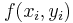. This Taylor series is

$y_{i+1}=y_{i}+f\left(x_{i}, y_{i}\right) h+f^{\prime}\left(x_{i}, y_{i}\right) \frac{h^{2}}{2}$

Expandingwith the chain rule, and substituting it back into the previous Taylor series expansion gives

$y_{i+1}=y_{i}+f\left(x_{i}, y_{i}\right) h+\left(\frac{\partial f}{\partial x}+\frac{\partial f}{\partial y} \frac{\partial y}{\partial x}\right) \frac{h^{2}}{2}$

The next step is to apply a Taylor series expansion to the k2 equation. The applied Taylor series expansion rule is

$g(x+r, y+s)=g(x, y)+r \frac{\partial g}{\partial x}+s \frac{\partial g}{\partial y}+\ldots$

and the resulting equation is

$f\left(x_{i}+p_{1} h, y_{i}+q_{11} k_{1} h\right)=f\left(x_{i}, y_{i}\right)+p_{1} h \frac{\partial f}{\partial x}+q_{11} k_{1} h \frac{\partial f}{\partial y}+O\left(h^{2}\right)$

where O(h2) is a measure of the truncation error between model and true solution.

When this Taylor series expansion result of the k2 equation, along with the k1 equation, is substituted into the general, and a grouping of like terms is performed, the following results:

$y_{i+1}=y_{i} \quad\left[a_{1} f\left(x_{i}, y_{i}\right) | a_{2} f\left(x_{i}, y_{i}\right)\right] h\left|\left[a_{3} p_{1}^{\partial f}\left|a_{s} q_{11} f\left(x_{i}, y_{i}\right)_{\partial y}^{\partial f}\right| h^{2} | O\left(h^{3}\right)\right.\right.$

Setting this result equal to the substituted general form will allow us to determine that, for these two equations to be equivalent, the following relationships between constants must be true.It should be noticed that these three equations, relating necessary constants, have four unknowns. This means that to solve for three constants, one must first be chosen. This results in a family of possible second-order Runge-Kutta methods.

## 6.10 Sage's Corner

Example of usage of Euler's Method:

Narrated example of using the Runge-Kutta Method: